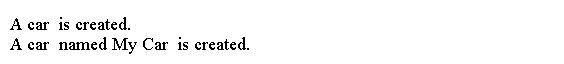Java 构造函数

声明构造函数

```<Modifiers> <Constructor Name>(<parameters list>) throws <Exceptions list> {

}
```

```public class Test {

public Test() {
// Code goes here

}
}
```

使用构造函数

new运算符创建一个对象，构造函数初始化该对象。

```Test t = new Test();
```

```class Cat {
public Cat() {
System.out.println("in constructor...");
}
}

public class Main {
public static void main(String[] args) {
// Create a Cat object and ignore its reference
new Cat();
// Create another Cat object and store its reference in c
Cat c = new Cat();
}
}
```重载构造函数

```class Car {
// Constructor #1
public Car() {
System.out.println("A car  is created.");
}

// Constructor #2
public Car(String name) {
System.out.println("A car  named " + name + "  is created.");
}
}

public class Main {
public static void main(String[] args) {
Car d1 = new Car(); // Uses Constructor #1
Car d2 = new Car("My Car"); // Uses Constructor #2
}
}
```从另一个构造函数调用构造函数

```class Test {
Test() {
this(1); // OK. Note the use of the keyword this.
}

Test(int x) {
}
}
```

从构造函数返回

```class Test {
public Test(int x) {
if (x < 0) {
return;
}

System.out.println("here");
}
}
```

构造函数的访问级别修饰符

```// Class Test   has  public access level
public class Test {
// Constructor #1 - Package-level access
Test() {
}

// Constructor #2 - public access level
public Test(int x) {
}

// Constructor #3 - private access level
private Test(int x, int y) {
}

// Constructor #4 - protected access level
protected Test(int x, int y, int z) {
}
}
```

默认构造函数